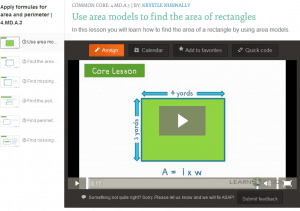# Applying Area and Perimeter Formulas for Rectangles in the Real World

##Measurement & Data – 4th Grade

#### Solve problems involving measurement and conversion of measurements.

CCSS.Math.Content.4.MD.A.3
Apply the area and perimeter formulas for rectangles in real world and mathematical problems. For example, find the width of a rectangular room given the area of the flooring and the length, by viewing the area formula as a multiplication equation with an unknown factor.

Teacher Notes
Reasoning about constructing situation equations for perimeter and area in real world and mathematical problems helps build an understanding of formulas.

Student Knowledge Goals
I can explain the area and perimeter formula.
I can use the formulas to solve problems.

Vocabulary
area
distance
formula
length
perimeter
product
rectangle
side
square unit
sum
two-dimensional figure
width

Lessons
Engage NY Module 3 A-2 – Solve multiplicative comparison word problems by applying the area and perimeter formulas.
Area and Perimeter Lessons

Student Video Lessons
Background Lessons
Virtual Nerd – Perimeter
Study Jams – Perimeter
Study Jams – Surface Area

Online Problems and Assessments
Khan Academy – Questions and Video Lessons
Perimeter
Area of squares and rectangles
Compare area and perimeter of two figures
Relationship between area and perimeter
Use area and perimeter to determine cost

Online Games
Perimeter and Area